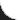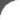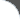Home | Tutorials | Articles | Videos | Products | Tools | Search
 Interviews | Open Source | Tag Cloud | Follow Us | Bookmark | ContactIn Browser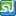StumbleUpon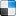del.icio.us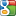Google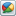Google Buzz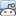reddit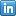LinkedIn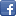Facebook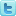TwitterLinkedin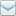E-Mail
 Tools and Libs > Uncommons Maths > Rational Numbers

# Rational Numbers

Uncommons Maths is a java library with nice math utilities and random number generators. This requires the library uncommons-maths-1.2.2.jar to be in classpath. The following example shows using Rational class.

 File Name  : com/bethecoder/tutorials/uncommons/RationalNumberTest.java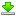Author  : Sudhakar KV Email  : [email protected]

 ``` package com.bethecoder.tutorials.uncommons; import org.uncommons.maths.number.Rational; public class RationalNumberTest {   /**    * @param args    */   public static void main(String[] args) {          Rational a = Rational.QUARTER;     Rational b = Rational.HALF;     Rational c = a.add(b);          System.out.println(a + "+" + b + " = " + c);               a = new Rational(1, 8);     b = new Rational(3, 8);     c = a.add(b);          System.out.println(a + "+" + b + " = " + c);          a = new Rational(4, 17);     b = new Rational(8, 13);     c = a.add(b);          System.out.println(a + "+" + b + " = " + c);   } }```

It gives the following output,
```1/4+1/2 = 3/4
1/8+3/8 = 1/2
4/17+8/13 = 188/221
```